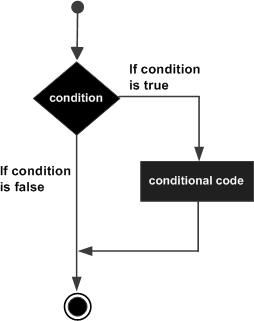# if statement in c

An if statement consists of a boolean expression followed by one or more statements.

### Syntax:

The syntax of an if statement in C programming language is:

```if(boolean_expression)
{
/* statement(s) will execute if the boolean expression is true */
}```

If the boolean expression evaluates to true, then the block of code inside the if statement will be executed. If boolean expression evaluates to false, then the first set of code after the end of the if statement(after the closing curly brace) will be executed.

C programming language assumes any non-zero and non-null values as true and if it is either zero or null, then it is assumed as false value.

### Flow Diagram:if statement

### Example:

```#include <stdio.h>

int main ()
{
/* local variable definition */
int a = 10;

/* check the boolean condition using if statement */
if( a < 20 )
{
/* if condition is true then print the following */
printf("a is less than 20\n" );
}
printf("value of a is : %d\n", a);

return 0;
}```

When the above code is compiled and executed, it produces the following result:

```a is less than 20;
value of a is : 10
```